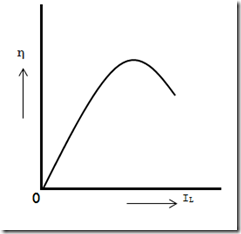# Condition for Maximum Efficiency in DC MachineThe DC generator efficiency is perpetual but varies with load. Think through a shunt generator supplying a load current IL at a terminal voltage V.
Then Generator output  =  VIL
Generator input  =  Output + Losses
=  VIL + Variable losses + Constant losses
=  VIL + I2a Ra + Wc
=  VIL + (IL + Ish2)Ra + Wc
( ∵  Ia = IL + Ish)

The shunt field current Ish is generally small as compared to IL and, therefore, can be neglected.
Generator input   =  VIL + I2a Ra + Wc

### Now                Efficiency   η  =  output / Input

=  VIL / (VIL + I2a Ra + Wc
=  1 / {1+[(ILRa/V)+(Wc/VIL)]}
The efficiency is always maximum when the denominator of Eq.(1) is minimum i.e.,
d/dIL {( ILRa/V) + (Wc+VI2L)} =0
Or
(Ra/V) – (Wc/ VI2L) =0
Or
Ra/V = Wc/VI2L
Or
I2LRa = Wc

I.e. Variable loss = Constant loss (IL ≈ Ia)
The load current corresponding to maximum efficiency is given by;
IL = √ Wc/Ra

Therefore, the efficiency of a DC generator will always be maximum once the load current is such that variable loss is the same to the constant loss. The fig shows the deviation of efficiency with load current.2 CommentsComments

#### Read Comment Policy We have Zero Tolerance to Spam. Chessy Comments and Comments with Links will be deleted immediately upon our review.

1.This comment has been removed by the author.

2.This site is good